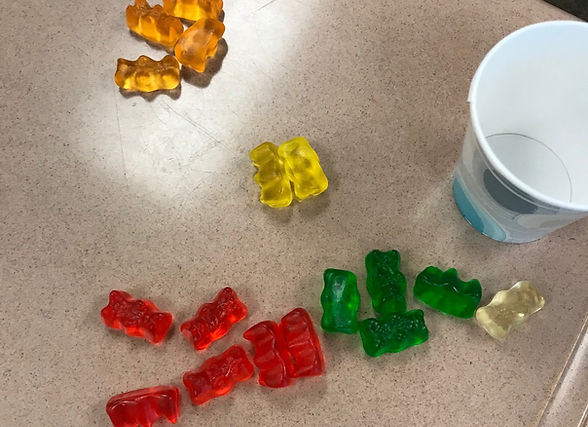## Chapter 12 - Day 4

##### Learning Targets
• State appropriate hypotheses and compute the expected counts and chi-square test statistic for a chi-square test based on data in a two-way table.

• State and check the Random, 10%, and Large Counts conditions for a chi-square test based on data in a two-way table.

• Calculate the degrees of freedom and P-value for a chi-square test based on data in a two-way table.

• Perform a chi-square test for homogeneity.

##### Activity: Does Gummy Bear Brand Matter?

Everyone knows that Haribo gummy bears are the best. Seriously. But we wondered if the distribution of color was the same for the off-brand. So we took a random sample of Haribo gummy bears and a random sample of “Meijer” gummy bears and compared the distribution of color.##### Chi-square GOF or Chi-square Test for Homogeneity?

This Activity may look similar to the M&M activity from the previous lesson, but there is a very important distinction. With the M&M activity, we were comparing data from one sample to a claimed distribution of color. With this gummy bear activity we are comparing data from one sample to data from another sample. This is analogous to the difference between a one-proportion z-test and a two-proportion z- test.

##### Chi-sure Test for Homogeneity or Chi-square Test for Independence?

Tomorrow, we will do a chi-square test for independence. The mechanics of this test are identical to the mechanics for the chi-square test of homogeneity. The difference is that a chi-square test for homogeneity has 2+ populations (Haribo, Meijer) and measures 1 categorical variable (color). For a chi-square test for independence, there is 1 population (senior students) and measures 2 categorical variables (gender, favorite class).